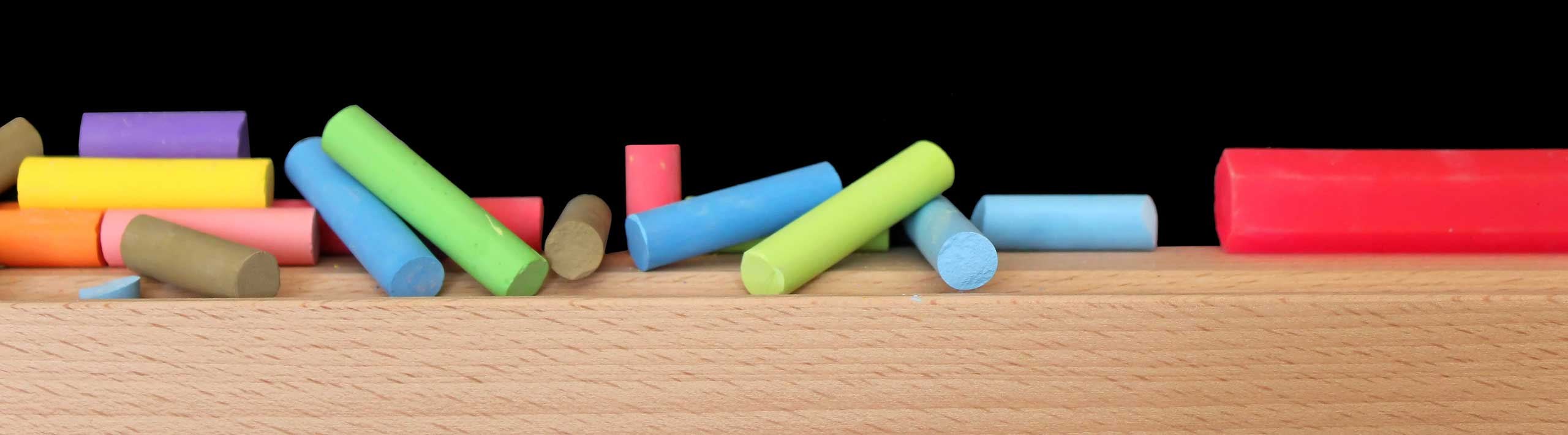# Answers to Polynomial and Rational Inequalities Questions

Answers to the questions in Polynomial and Rational Inequalities - Questions and Answers are presented here.

 Question 1: Solve the inequality.-(x + 2) + 2x > 2(x - 3) + 3x answer e: (-infinity , 1) Question 2: Solve the polynomial inequality. (6x + 2) (10 - 20x) > = 0 answer e: [-1/3 , 1/2] Question 3: Solve the inequality. x2 + 3x - 4 > 0 answer b: (-infinity , -4) U (1 , +infinity) Question 4: Solve the inequality. 2x2 < 32 answer a: (-4 , 4) Question 5: Solve the inequality. 25x2 <= 9x answer d: [0 , 9/25] Question 6: Solve the inequality. (1 + x) / |x - 2| > 0 answer b: (-1 , 2) U (2 , +infinity) Question 7: Solve the inequality. (2x2 + 3) (x + 2) / (x2 - 4) > = 0 answer c: (2, +infinity) Question 8: Solve the inequality. 4 / (6x - 4) < = 2 / (2x + 2) answer d: (-1 , 2/3) U [4 , +infinity) Question 9: Solve the inequality. 2 < |x + 1| / 2 - 2 < 5 answer c: (-15, -9) U (7 , 13) Question 10: Solve the inequality. x2 < -x4 answer a: no solutions Solve Inequalities in One Variable